# Kerala Syllabus 10th Standard Chemistry Solutions Chapter 2 Gas Laws Mole Concept

You can Download Gas Laws Mole Concept Questions and Answers, Summary, Activity, Notes, Kerala Syllabus 10th Standard Chemsitry Solutions Chapter 2 help you to revise complete Syllabus and score more marks in your examinations.

## Kerala State Syllabus 10th Standard Chemistry Solutions Chapter 2 Gas Laws Mole Concept

### Gas Laws Mole Concept Text Book Questions and Answers

Gas Laws And Mole Concept Questions And Answers Kerala Syllabus 10th Text Book Page No: 33

→ Complete the table 2.1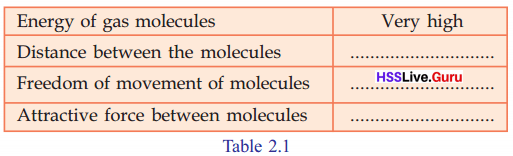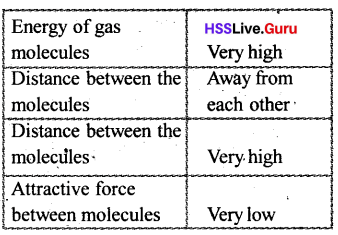→ If a gas which is kept in a cylinder having a volume of 1 liter, is completely transferred to another 5-liter cylinder then what will be the volume of the gas?
5 liter

→ Press the piston after closing the nozzle of the syringe. What will happen to the volume of air inside the syringe?
Volume decreases

→ Explain it on the basis of the distance between the molecules of gas and their freedom of movement?
Gases molecules are separated from each other by a large distance. As a result, there will be a lot of vacant spaces. So as the piston is pressed, the molecUles come closer and this volume decreases

Use the Molarity Calculator Chemistry to calculate the mass, volume or concentration required to prepare a solution of compound of known molecular weight.

Sslc Chemistry Chapter 2 Kerala Syllabus  Text Book Page No: 34

→ What is the specialty of the movement of the molecules?
Molecules move in all possible directions

→ What assumption can be made regarding the possibility of collision between gas molecules?
The molecules collide each other.

→ Which energy gained due to the movement of molecules? Potential energy/Kinetic, energy.
Kinetic energy→ When a gas is heated, temperature is increased. What happens to the movement of molecules if the temperature of the gas is increased?
Speed of motion increased

→ Asa result, what happens to the energy of the molecules?
Energy of molecules increase

→ Volume
The space needed for a substance to occupy is its volume. The volume of solids and liquids are definite. But the volume of a gas is the volume of its container in which it is present.

→ Pressure
The force exerted at unit area is pressure, Therefore, force at unit area/ pressure
$$=\frac{\text {Force exerted at the surface}}{\text {Area of the surface}}$$

→ Temperature
The average Kinetic energy of all the molecules in a substance is its temperature

Sslc Chemistry Chapter 2 Questions And Answers Text Book Page No: 35→ Is there any change in the number of molecules?
No

→ What happens to the pressure when the volume is decreased?
Pressure increases

→ What is the specialty of the movement of the molecules?
Molecules move in all possible directions

Mass to Moles Calculator — The quantity of substance n in moles is equal to the mass m in grams divided by the molar mass M in g/mol.→ What changes can you observe in the volume of the gas inside the syringe?
Volume is formed to be decreasing.

→ What about decreasing the pressure?
Volume increasing

→ What relation do you arrive at between pressure and volume of the gas?
As pressure increases volume decreases when pressure is reduced volume increased.

Gas Laws And Mole Concept Kerala Syllabus 10th Text Book Page No: 36→ The size of the air bubbles rising from the bottom of an aquarium increases. Can you explain the reason?
As the bubbles move upward, the pressure on them decreases. This causes increase in volume. So as the bubbles move upward, their size increases.

→ What do you observe?
Ink rises through the tube→ What is the reason for the rising of the ink upwards?
When the bottle is placed in hot water, air inside the bottle becomes hot. This causes expansion of air. This pushes ink in the tube. So ink rises through the tube.

→ What did you observe on cooling the bottle after taking it out? Why?
Ink comes down. Because as air becomes cool, Its volume decreases.

→ What can you infer about the relation between the volume and temperature of a gas?
When temperature is increased volume of gas increases. Similarly, when temperature is decreased, The volume decreases.

→ Complete the table 2.2Sslc Chemistry Chapter 2 Notes Kerala Syllabus Text Book Page No: 37

→ In which unit is the temperature stated?
Kelvin (K)

→ What happens to the volume when the temperature is increased?
Volume increases.

→ If an inflated ballon is kept in sunlight, it will burst. What may be the reason for this?
When the ballon placed in sun light, temperature increases. so volume of air inside the ballon increases. Thus ballon expands and finally bursts.

→ What happens to the volume of the gas when its pressure is decreased or temperature is increased. volume increased/decreased.
volume increased→ If the temperature and pressure are kept constant how can we increase the volume?
Fill some more gas.

→ Fill the cylinder with a little more gas. Does the number of molecules increase or decrease now?
Then number of molecules increases

→ What is the relation between the volume and number of molecules?
When the number of molecules increases, volume increases.

→ According to Avagadro’s law when the temperature and pressure remain constant on which factor does the volume of gas depend?
Depends on the number of molecules.

Kerala Syllabus 10th Standard Chemistry Chapter 2 Text Book Page No: 38

→ If the mass of a coin 5g, then what will be the mass of thousand coins?
5 x 1000 = 5000g

→ If the mass of coins in a bag is 50,000 g, then how many coins will be there?
$$\frac { 50000 }{ 5 }$$ = 10000

→ Like this we can calculate the number of coins on the basis of mass. Can‘t we?
Yes, it becomes easy.

→ Is their any relation between the mass and the number, if the particles are of the same mass.
Yes→ What may be the method of stating the mass of atoms?
The mass of an atom is compared to the mass, of another atom and expressed as a number which shows how many times it is heavier than the other atom. The atomic mass of elements are expressed by considering 1/12 mass of an atom of carbon-12 as one unit.

→ What do you understand from the statement that the atomic mass of Helium is 4?
Atomic mass of Helium is 4. That is mass of one atom of Helium is 4 times of 1/12th mass of carbon atom.

Enter the formula and press “calculate” to work out the molar mass calculator with steps, the number of moles in 1 g and the percentage by mass of each element.

Chemistry Class 10 Chapter 2 Kerala Syllabus Text Book Page No: 39

→ How many oxygen atoms combine with one carbon atom?
2 Oxygen atoms

→ How many oxygen atoms combine with 1000. carbon atoms?
2000 oxygen atoms.

→ How many atoms are present in 12g carbon?
2 × 6.022 × 1023 oxygen atoms

→ What will be the mass of these tabs ?
2 × 16 = 32g

Gas Laws And Mole Concept Extra Questions 10th Text Book Page No: 40

→ Complete the table 2.5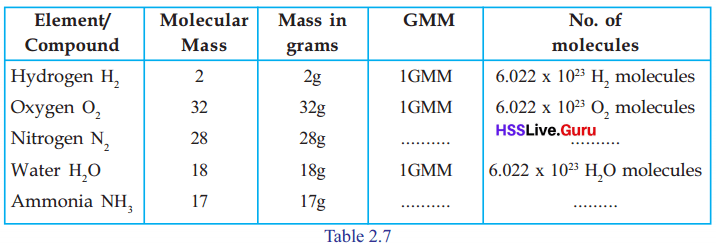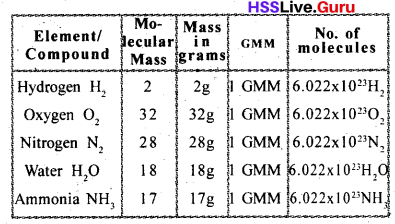→ 1GAM sodium means 23g sodium. This contains 6.022 × 1023 atoms. If so, how many GAM is present in 69 g sodium? How many atoms are present in it?
$$\frac { 69 }{ 23 }$$ = 3 GAM,
3 × 6.22 × 1023 sodium atoms.

Gas Laws And Mole Concept Notes Pdf Kerala Syllabus 10th Text Book Page No: 41

→ How many GAMs are present in each the samples given below? Calculate the, number of atoms present in each of die sample ? (Atomic mass N= 14, O= 16)
1. 42g Nitrogen,
2. 80g Oxygen
1. 42g Nitrogen:
No.of GAM = $$\frac { 42 }{ 14 }$$ = 3GAM
No.of atoms = 3 × 6.022 × 1023

2. 80g Oxygen:
No.of GAM = $$\frac { 80 }{ 16 }$$ = 5GAM
No.of atoms = 5 × 6.022 × 1023

→ Complete the table 2.6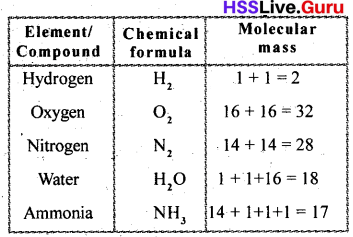→ Calculate the molecular mass of glucose (C6 H12 O6) and sulphuric acid (H2 SO4)
Molecular mass of glucose
= 6 × 12 + 12 × 1 + 6 × 16 = 72 + 12 + 96 = 180 g
Molecular mass of sulphuric acid
2 × 1+ 1 × 32 + 4 × 16 = 2 + 32 + 64 = 98 g

Gas Laws And Mole Concept Questions And Answers Pdf 10th Text Book Page No: 42

→ Complete the table 2.7→ What is the molecular mass of oxygen?
32 g

→ How many GMM is present in 32g oxygen?
1 GMM

→ How many molecules are present in it?
6.022 × 1023 oxygen molecules→ How many GMM is present in 28 gm nitrogen?
1 GMM

→ How many molecules are present in N2?
6.022 × 1023 N2, molecules

→ How many GMM is present in 18 gm water?
1 GMM

→ How many H2O molecules arf present in it?
6.022 × 1023 H2O Molecules

→ Calculate the number of GMM present in 96g oxygen?
$$\frac { 96 }{ 32 }$$ = 3GMM

Sslc Chemistry Chapter 2 Notes Pdf Kerala Syllabus Text Book Page No: 43

How many GMM are present in each of the given samples? Calculate the number of molecules present in each sample ?

→ 360 g glucose (Molecular mass = 180)
No.of GMM = $$\frac { 360 }{ 180 }$$ = 2 GMM
No. of molecules = 2 × 6.022 × 1023

→ 90g water (Molecular mass = 18)
No.of GMM = $$\frac { 90}{ 18 }$$ = 5 GMM
No. of molecules = 5 × 6.022 × 1023

→ How many molecules of water are present . in one mole of water ?
6.022 × 1023 water molecules→ What is its mass?
18 g

→How many GMM is present in it?
1 GMM

Sslc Chemistry 2nd Chapter Notes Kerala Syllabus Text Book Page No: 44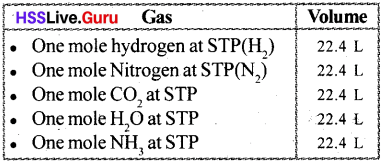22.4 L of a gas at STP = 1 mole
44.8 Lofa gas at STP = = 2 mole
224 L of a gas at STP = $$\frac { 224 }{ 22.4 }$$ = 10 mole

Gas Law And Mole Concept Kerala Syllabus 10th Text Book Page No: 45

Complete the flow chart given below, related to one mole of substance.### Gas Laws Mole Concept Let Us Assess

Gas Laws And Mole Concept Notes Kerala Syllabus 10th Question 1.
Examine the date given in the table (Temperature and number of molecules of the gas are kept constant).
a. Calculate P × V
b. Which is the gas law related to this?
a. 8L atm
b. Boyle’s law

Gas Laws And Mole Concept Pdf Kerala Syllabus 10th Question 2.
Analyse the situations given below and explain the gas law associated with it.
a. When an inflated balloon is immersed in water, its size decreases.
b. A balloon is being inflated
b. Boyle’s law

Gas Laws And Mole Concept Class 10 Kerala Syllabus Question 3.
Certain data regarding various gases kept under the same conditions of temperature and pressure are given below.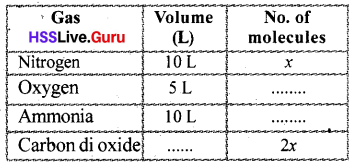a. Complete the table?
b. Which gas law is applicable here?
a.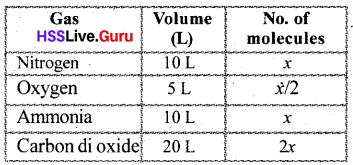Hss Live Guru 10th Chemistry Kerala Syllabus Question 4.
a. Calculate the mass of 112 L CO2 gas kept at STP (molecular mass = 44)
b. How many molecules of CO2 are present in it?Sslc Chemistry Chapter 2 Gas Laws And Mole Concept Question 5.
Calculate the volume of 170g of ammonia at STP ? (Molecular mass 17)
Number of moles = $$\frac { Given mass }{ GMM }$$ = $$\frac { 170 }{ 17 }$$ = 10 moles
Volume at STP = mole × 22.4 L = 10 × 22.4 L = 224 L

Hsslive Chemistry 10th Kerala Syllabus Question 6.
Find out the number of moles of molecules present in the samples given below (GMM-N2=28g, H2O= 18g)
a. 56g N2
b. 90g H2O
a. Number of mol molecules = $$\frac { Mass }{ GMM }$$ = $$\frac { 56 }{ 28 }$$ = 2

b. Number of mol molecules = $$\frac { 90 }{ 18 }$$ = 510th Chemistry 2nd Chapter Kerala Syllabus Question 7.
The molecular mass of ammonia is 17.
a. How much is the GMM of ammonia?
b. Find out the number of moles of molecules present in 170g of ammonia.
c. Calculate the number of ammonia molecules present in the above sample of ammonia?
a. GMM of ammonia (NH3) = 14 + 3 × 1 = 17g = 1GMM

b. Number of mole molecules = $$\frac { Mass }{ GMM }$$ = $$\frac { 170 }{ 17 }$$ = 10

c. Number Of molecules = Mole × 6.022 × 1023
= 10 × 6.022 × 1023

Class 10 Chemistry Chapter 2 Kerala Syllabus Question 8.
The molecule’s mass of oxygen is 32.
a. What is the GMM of O2
b. How many moles of molecules are there in 64g of oxygen? How many molecules are there in it?
c. Calculate the number of oxygen atoms present in 64g of oxygen?
a. GMM of O2 =2 × 16 = 32 g

b. No. of mole molecules = $$\frac { Mass }{ GMM }$$ = $$\frac { 64 }{ 32 }$$ = 2
Number of molecules = mole × 6.022 × 1023
= 2 × 6.022 × 1023
c. c. Number of atoms = Number of molecules × number of atoms in one molecules Number of atoms in one molecules of oxygen (O2) =2
∴ total number of atoms = 2 × 6.022 × 1023 × 2
=4 × 6.022 × 1023

### Gas Laws Mole Concept Extended activities

Hss Live Guru Chemistry 10 Kerala Syllabus Question 1.
How many grams of carbon and oxygen are required to get the same number of atoms as in one gram of Helium?
GAM of Helium = 4 g
Number of mole atoms in 4 g of Helium = 6.022 × 1023
Number of atoms in 1 gofHelium = $$\frac { 1 }{ 4 }$$ × 6.022 × 1023
GAM ofCarbon = 12 g
∴ Number of atoms in 12gofCarbon=6.022 x 1023
∴ Mass required for $$\frac { 1 }{ 4 }$$ × 6.022 × 1023
Carbon atoms = $$3 \mathrm{g}\left(12 \times \frac{1}{4}\right)$$
GAM of Oxygen = 16 g.
∴ Number of atoms in 16 g of Oxygen = 6.022 × 1023
∴ Mass required for $$\frac { 1 }{ 4 }$$ × 6.022 × 1023
Oxygen atoms = $$4 \mathrm{g}\left(16 \times \frac{1}{4}\right)$$Kerala Syllabus 10th Standard Chemistry Guide Question 2.
Examine the samples given:
a. 20 g of He
b. 44.8 L of NH3 at STP
c. 67.2 L of N2 at STP
d. 1 mol of H2SO4
e. 180 g of water.
i. Arrange the samples in increasing order of the number of molecules in each.
ii. What will be the ascending order of the total number of atoms?
iii. What will be the masses of samples b, c, and d?Mole Concept Class 11 Question 3.
In 90 grams of water.
a. How many molecules are present?
b.What will be the total number of atoms?
c. What will be the total number of electrons in this sample?
a. GMM= 18 g
∴ Number of molecules
$$\frac { 90 }{ 18 }$$ × 6.022 × 1023 = 5 × 6.022 × 1023 18

b. ∴ Number of atoms = 3 × 5 × 6.022 × 1023 = 15 × 6.022 × 1023

c. Electrons in 1 H atom = 1
Electrons in 1 O atom = 8
Total electrons in H2O molecule? 10
Total electrons in 90 g H2O = 10 × 5 × 6.022 × 1023 = 50 × 6.022 × 1023

### Gas Laws Mole Concept Orukkam Questions and Answers

Question 1.
a. Complete the table based on the data given in the box.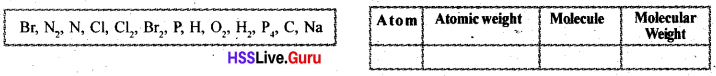b. Express atomic weight and molecular weight in grams. How many moles is this? Find out the number of Atoms or molecules in it?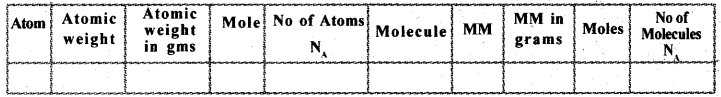a.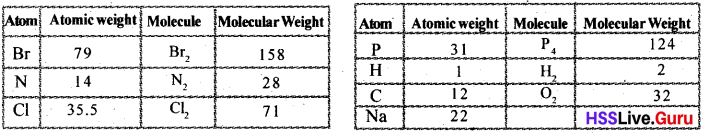b.Question 2.
Complete the table based on the molecules given in the first column and then answer the question given below.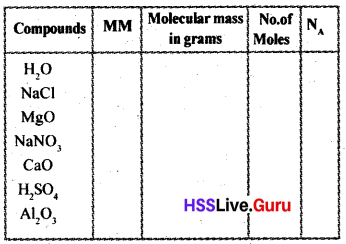a. 10 Mole of water = …………. g …………… Molecules
5 mole of CaO = ……….. g ………… Molecules
2 Mole of H2SO4 = …………. g ………… Molecules
1/2 Mole of Al2O3= …………. g ………….. Molecules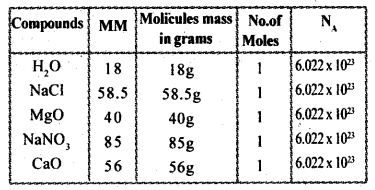a. 10 Mole of water = 10 × 18 = 180g, 10 × 6.022 × 1023 Molecules
5 mole of CaO = 280g, 5 × 6.022 × 1023 Mol-ecules
2 Mole of H2SO4 = 196g, 2 × 6.022 × 1023 Mol-ecules
2- Mole of AlO = 100g, 1/2 × 6.022 × 1023

Question 3.
Complete the table.Question 4.
Complete the data.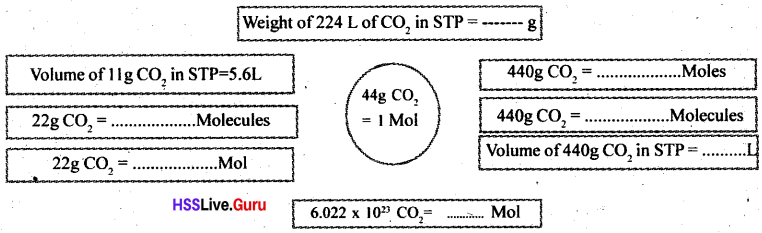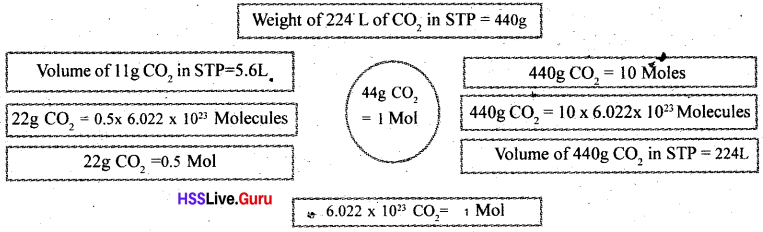Question 5.
Based on the reaction given below, write the answers for the questions.
N2 + 3H2 → 2NH3 ;
a. Write the ratio of reactant molecules and product molecules.
b. How many moles of Ammonia forms when we take 2 moles of Nitrogen and six moles of Hydrogen?
c. Two moles of Nitrogen and three moles of hydrogen are taken in jar? Will they react together?
d. How many moles of Nitrogen and Hydrogen is needed for rearing 20 moles of Ammonia?
a. 1:3:2
b. 2 Mole
c. No
d. 10 Mole Nitrogen 30 Mole HydrogenQuestion 6.
Balance the given equation and then write down the answers for the questions given below.
CH4+O2 → CO2 + H2O
a. How many moles of C02 formed when 20 moles of Methane burns in air?
2C2H6 + 7O2 → 4CO2 + 6H2O
b. Based on the equation above, How many moles of CO2 is formed when 10 moles of Ethane is burned in air ?
a. When 1 mole of methane bum in air 1 mole of CO2 gas is formed.
When 20 moles methane burn 20
moles of CO2 are formed.
The molecular weight of 20 moles = 20 × 44 = 880 g

b. When 2 moles of ethane is burned 4 moles of CO2 is formed.
The number of moles of CO2 when 1 mole of ethane is burned = 4/2 = 2 mole.
The number of moles when 10 moles of ethane bums = 2 x 10 = 20 mole.
Weight of 20 moles = 20 × 44 = 880 g

Question 7.
Based on the given equation write down the answers.
2H2+ O2 → H2O
a. How much Oxygen and Hydrogen is needed for making 1800g of water vapor?
b. How many moles of Oxygen is needed for the reaction with one mole of Hydrogen?
a. 36 g of water vapour can be made using 4 g hydrogen.
The amount of hydrogen required to make 1 g water vapour = 4/36
The amount of hydrogen required to produce 1800 g watervapour= 4/26 × 1800 = 200 g
Mass of oxygen = 1800 – 200 = 1600 g

b. 0.5 Mole

### Gas Laws Mole Concept Evaluation Questions

Question 1.
Find out the number of moles of hydrogen and Oxygen atoms present in 10 moles of HCI.
One mole HC1 contains 1 mole of hydrogen and 1 mole of chlorine.
Hydrogen contained in 10 moles of HC1 = 1 × 10 =10 mol,
Clatom = 10 × 1 = 10 mol

Question 2.
Find out the mass of Hydrogen atom and chlorine atom in 10 moles of HCI.
Mass of 10 mole hydrogen atom = 10 × 1 = 10g
Mass of 10 mole chlorine atom =10 × 35.5 = 355 g.

Question 3.
a Find out the mass of one mole of CaCO3. How many moles of calcium present in 1000g CaCO3?
b. How many moles of Oxygen present in 1000gms of CaCO3?
a. Mass of 1 mole of CaCO3 = 40 + 12 + 48 = 100 gram.
No of moles ofCa in 1000 g CaCO3 = $$\frac { 1000 }{ 100 }$$ = 10 mol

b. No of moles of Ca in 10 moles CaCO3 =10 × 1 = 10 mol
No of moles of oxygen in 10 moles of CaCO3 = 10 × 3 = 30mol.This Combined Gas Law Calculator can help you estimate either the pressure, temperature or the volume of gas.

Question 4.
Find out number of moles of water formed when 4gms of Hydrogen and 32 gms of Oxygen combined together. What is the result when 5 gms of Hydrogen and 32 gms of Oxygen combined together?
Ans. When 4 g of hydrogen and 32g of oxygen are combined 36 g of water $$\frac { 37 }{ 18 }$$ = 2 mol
5g H + 32 gO → 37g H2O
No of moles in 3 7 g of water = $$\frac { 37 }{ 18 }$$ = 2.055 mol

Question 5.
a How much grams of NaCl is needed for making 2 molar solution (NaCl – 58.5). Wh-at is the amount of water needed for this?
b. How will you change a two molar solution of Sodium Chloride into 5 major?
a. Mass of 2 moles of NaCl = 2 × 58.5 = 117 g
1 liter water is required for this.

b. When 2 moles of NaCl is dissolved in 4 liter of water 5 molar solution is obtained.

Finally, you encounter how to find molar concentration step-by-step manually, and if your preference indulges with instant calculations.

Question 6.
How many moles of Cl2 present in 11.2 L of same in STP? Find out the mass of this?
No.of moles present in 11.2 litre of chlorine = $$\frac { 11.2 }{ 22.4 }$$ = 0.5 mol
Mass of 0.5 moles of chlorine = 0.5 × 35.5 = 17.759 g.

Question 7.
Find out the mass of Oxygen atom in 44.8L of CO2 in STP.
No of moles in 44.8 litre of CO2 $$\frac { 44.8 }{ 22.4 }$$ 2 mol,
1 Mole of CO2 contains 1 mole of C and 1 mole of O2
∴ 2 Mole of CO2 contains 2 mole of O2 or 4 mole of oxygen atom.
Man of oxygen atom 4 × 16 = 64 g

Question 8.
Find out the amount of CO2 formed when the burning of one mole of Ethane.
2 moles of CO2 is formed when 1 mole of ethane burns.
Mass of 2 moles of CO2 = 2 × 44 = 88gQuestion 9.
Why are atomic mass of some elements are infractions ?
The atomic masses of some elements are infractions because they exist as a mixture of isotopes of different masses. The fractional atomic masses arise because of this mixture.
Average mass = $$\frac { Total mass of all atoms }{ numbers of atoms }$$

### Gas Laws Mole Concept SCERT Questions and Answers

Question 1.
One GAM substance contains Avogadro number of particles in it.
a. How many particles are there in Avogadro number ?
b. Write the number of atoms present in each of the following.
i. 32g Sulphur
ii. 32g Oxygen
iii. 32g Carbon
(Atomic mass S = 32, O = 16, C = 12)
a. 6.022 × 1023
b. i.6.022 × 1023
ii. 2 x 6.022 × 1023
iii $$\frac { 32 }{ 12 }$$ × 6.022 × 1023

Question 2.
a. Group the following into pairs having same number of atoms.
A. 2g Hydrogen
B. 16g Oxygen
C. 14g Nitrogen
D. 8g Helium (Atomic mass H=1, O= 16, N =14, He=4)
b. How many atoms are present in each pair?
a. A, D2g Hydrogen, 8g Helium
B, C 16g Oxygen, 14g Nitrogen

b. A, D – 2 × 6.022 × 1023
B,C – 6.022 × 1023

Question 3.
N2 + 3H2 → 2NH3
a. What is the ratio between the reactant molecules in the above reaction?
b. Complete the following table.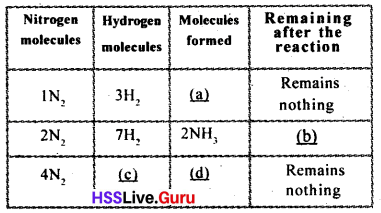a. 1:3
b. a – 2 NH3,
b – l H2,
c – 12H2,
d – 4NH3

Question 4.
2H2 + O2 → 2H2O
a. What is the ratio between the reactant molecules in the above reaction?
b How many O2 molecules are required to react 100 H2 molecules completely?
c. How many water molecules are formed when 1000 H2 molecules are reacted completely
a. 2:1
b. 50 O2 molecules
c. 1000 H2O molecules

Question 5.
Complete the following table. (All the elements given are diatomic. Atomic mass O=16, N=14, CI=35.5)a. 6.022 × 1023
b. 6.022 × 1023
c. 71 g
d. 14 g

Question 6.
A sample of substances are given.Hint: Molecular mass NH3 = 17, N2 = 28, H2SO4 = 98, O2=32
a. Which of these samples have same number of molecules?
b. Which of these samples has least number of molecules?
a. 68g NH3, 128gO3
b. 49 g H2SO4Question7.
Pick out the correct statements from the following. Also, correct the incorrect statements.
a The number of molecules present in 1 mol hydrogen and 1 mol oxygen are same,
b. 2 mol chlorine contains 4 x 6.022 x 1023 chlorine molecules.
c. The mass of 1/2 mol nitrogen gas is 14 g.
d. 0.5 mol water has the mass 9g. There are 6.022 × 1023 H20 molecules in it. (Atomic mass H = 1, O = 16, CI= 35.5, N= 14)
Correct statements – a, c
No. of molecules in 2 mol chlorine is 2 × 6.022 × 1023
Mass of 0.5 mol water is 9g. So it contains 0.5 × 6.022 × 1023 H2O molecules.

Question 8.
Complete the following.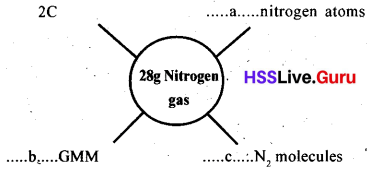a. 2 × 6.022 × 1023
b. 1GMM
c. 6.022 × 1023

Question 9.
67.2 L of Carbon dioxide gas is filled in a cylinder at STP.
a. Calculate the mass of CO2 present in it. (Atomic mass- C = 12, O = 16)
b. Calculate the number of molecules present in the cylinder.
a. Molecular mass of CO2=12 × 1+16 × 2 = 12 + 32 = 44
No. of moles in 67.2L CO2 at STP = $$\frac { 67.2L }{ 22.4L }$$ = 3
b. 3 × 6.022 × 1023

Question 10.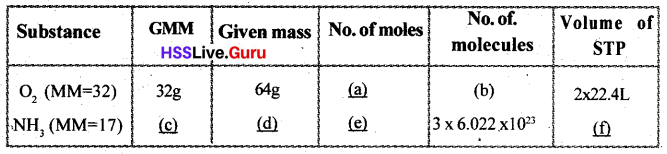a. 2
b. 2 × 6.022 × 1023
c. 17 g
d. 51 g
e. 3
f. 3 × 22.4 L

Question 11.
CH4 + 2O2 → CO2 + 2H2O
The equation describes the combustion of methane in air.
a. How many moles of oxygen is required for the complete combustion of 16g CH4?
b. Calculate the amount of CO2 formed when 100g of CH4 is completely burnt?
a. 2 mol
b. Amount of CO2 produced by the combustion of 16g CH4 = 44g
Amount of CO2 produced by the combustion of 1 gm CH4 = $$\frac { 44 }{ 16 }$$ g
Amount of CO2 produced by the combustion of 100g CH4 = $$\frac { 44 }{ 16 }$$ × 100gQuestion 12.
45 g glucose is taken in a beaker and made into 1 L (MM = 180).
a. Calculate the molarity of the solution,
b. Above solution is made up to 2 L by adding more water. What will be the molarity of the resultant solution?
c. How will you prepare IM solution of glucose with the same quantity (45 g) of glucose?
a. 0.25
b. M = $$\frac { n }{ v }$$ = $$\frac { 0.25 }{ 2 }$$
c. Add 250 ml water in 45 g glucose

Question 13.
Two gases occupy equal volume at STP are shown below.
(Atomic mass S = 32, O =16, N = 14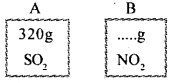a. Find the mass of the gas in B.
b. Calculate the number of molecules present in B.
a. No. of moles in 320g SO2 = $$\frac { 320 }{ 64 }$$ = 5
Mass of 5 mol NO2 = 5 × 46 = 230 g
b. No.of molecules present in B = 5 × 6.022 × 1023

Question 14.
The balanced chemical equation of a reaction (at STP) is given below.
2H2(g) + O2(g) → 2H2O(g)
a. Calculate the volume of oxygen required to combine completely with 224 L of the hydrogen at STP.
b. Calculate the mass of water formed as a result of the reaction (a).
a. 112 L
b. Volume of water obtained when 224 L hydrogen completely reacts with oxygen = 224 L
No. of moles in 224 L water = $$\frac { 224 }{ 22.4 }$$ = 10
Massof 10 mol water = 10 × 18 = 180 g

Question 15.
Complete the table.Hint: (MM – CO2 = 44, CH4 = 16, SO2 = 64)
a. 67.2 l
b. 132 g
c. 1/4.
d. 4g
e. 11.2 L
f. 1/2.

Question 16.
Analyse the following equation
2NO(g) + O2(g) → 2NO2(g)
a. Calculate the number of the moles of NO required to combine completely with 112 L of Oxygen at STP.
b. Calculate the mass of NO2 formed when 112L of oxygen reacts completely?
a. 10 mol
b. 2NO(g) + O2(g) → 2NO2(g) (2 : 1: 2)
No. of moles in 112L O2 = 5 mol
According to equation no. of moles of NO2 obtained by reacting oxygen completely with nitric oxide = 2
No. of moles of NO2 obtained by reacting 5 mol oxygen completely = 10
Massof 10 mol NO2 = 10 × 46 = 460gQuestion 17.
The chemical equation of the decomposition of calcium carbonate is given below.
CaCO3→ CaO + CO2
(HintMM: CaCO3 – 100, CaO – 56, CO2 – 44)
a. Calculate the mass of CaCO3 required to get 224 g of CaO?
b. Calculate the number of CO2 molecules fronted when 224g of CaO is obtained?
a. CaCO3 → CaO + CO2
100g 56g 44g
I I : I
Amount of CaCO3 required to get 56g of CaO = 100g
Amount of CaCO3 required to get 1 g of CaO = $$\frac { 100 }{ 56 }$$
Amount of CaCO3 required to get 224 g of CaO = $$\frac { 100 }{ 56 }$$ × 224 = 400g
b. 4 × 6.022 × 1023

Question 18.
You are requested to make 20 moles of NaCl into packets of 100g each. (Hint^Molecular mass of NaCl is 58.5)
a. How many packets of NaCl can be prepared?
b. Is there any NaCl remaining? If so, how much?
a. Mass of 20 mol NaCl = 20 × 58.5 = 1170g
1170 g NaCl can be made into 11 packets with 100g each.
b. Remaining NaCl = 1170 – 1100 = 70g

### Gas Laws Mole Concept Exam Oriented Questions and Answers

Very Short Answer Type Questions (Score 1)

Question 1.
GAM of Hydrogen is 1 g.
a. How many number of atoms are there in 1 g of Hydrogen?
b. Find the mass of 1 atom of hydrogen.
a. 1g hydrogen = 6.022 × 1023 atoms
b. Mass of 1 atom of hydrogen
= $$\frac{1 g}{6.022 \times 10^{23}}$$ = 1.66 × 10-24 g

Question 2.
Number of molecules of substance is 3.011 × 1024.
a. What is the number of molecules of 1 mole of any substance?
b. Find the number of moles of 3.011 × 1024 molecules.
a. 6.022 ×1023
b. Number of moles of molecules = $$\frac { Number of molecules }{ NA }$$
= $$\frac{3.011 \times 10^{24}}{6.022 \times 10^{23}}=5$$

Short Answer Type Questions (Score 2)

Question 3.
Fill the patterns.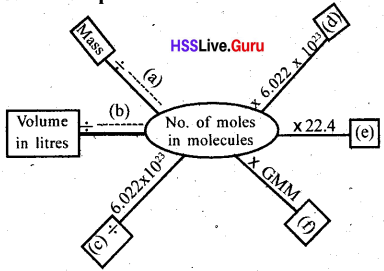a. GMM
b. 22.4
c. Number of molecules
d. Number of molecules
e. Volume in litres
f. Mass

Question 4.
Identify the incorrect statements from those given with respect to the arrangements of molecules in gases.
a. The minute molecules are present without any freedom of movement
b. Collision take place between the molecules,
c. Increasing the number of molecules at constant volume causes the decrease in number of collisions.
d. The energy of molecules are comparatively high.
a. The statements (a) and (c) are not correctQuestion 5.
The pressure of 20L of a gas kept at 300 K is found to be 2 atoms. If the pressure is increased to 3 atom at the same temperature, what will be the new volume?
According to Boyles law, PV = a constant
Therefore, P1 V1 = P2 V2
Here, P1 = 2atm V1 = 20L P2 = 3atm V2=?
∴ 2 × 20 = 3 × V2
Thus, V2 = $$\frac { 2 × 20 }{ 3 }$$ = 13.3 L

Charles Law Calculator is a free online tool that displays the volume of gas that tends to expand when heated.

Question 6.
If the temperature of 5L of a gas at atmospheric pressure is changed from 200K to 50 K, what will be the volume?
According to Charles law, $$\frac { V }{ T }$$ = a constantQuestion 7.
What will be mass of 89.6 L of ammonia (NH3) gas at STP?
89.6 L of NH3 gas at STP = $$\frac { 89.6 }{ 22.4 }$$ = 4 mol
GMM of NH3 = 14 + 3 = 17g
Mass = mole × GMM = 4 × 17 = 68g

Question 8.
8. a. What is molar volume?
b. What is the molar volueofa gas at STP?
a. The volume of one mole of a gas is called molar volume,
b. 22.4 L

Question 9.
Look at the balanced equation given.
2NaOH + CO2 → Na2 CO3 + H20
a. Find out the mass of NaOH needed for 264 g CO2 to react completely.
b. Find out the total number of moles of water molecules when CO2 reacts.
a. GMM of CO2 = 44 g
∴Number of moles in the molecule of 264 g CO2 = $$\frac { 264 }{ 44 }$$ = 6
According to the equation NaOH needed for the reaction of 1 mole CO2= 2 moles
∴ NaOH needed for the reaction of 6 moles CO2 = 2 × 6 = 12 moles
GMM of NaOH = 23 + 16 + 1 = 40 g
Total mass of NaOH = 12 × 40 = 480 g

b. H2O formed when lmole CO2 reacts = 1 mole
∴ Total number of moles of water molecules when 6 mole CO2 reacts = 6 molesQuestion 10.
2C4H10 + 13O2 → 8CO2 + 10H2O
This is the equation of ignition of cooking gas butane.
Calculate the volume of CO2 in STP during the complete ignition of 14 kg of cooking gas.
Mass of Butane (C4H10) = 14 kg = 1400g
GMM of C4H10 = 4 × 12 + 10 × 1 = 58 g
∴ Number of moles in molecules = $$\frac { 1400 }{ 58 }$$ = 241.38
Amount of CO2when 2 moles of C4 H10 ignites = 8 moles of C4H10 ignites = $$\frac { 8 }{ 2 }$$ × 965.52 moles
∴ Volume of CO2 formed in STP
= 965.52 × 22.4 L = 21627.65 L

Question 11.
Write down the preparation of 100 ml NaOH solution of 0.1 M.
GMM of NaOH = 40 g
Molarity = $$\frac{\text { Number of moles of solute }}{\text { Volume of solution in litres }}=\frac{\mathbf{n}}{\mathbf{v}}$$
M = 0.1 V=100ml = 0.1 L
0.1 = n/0.1
∴ n = 0.1 × 0.1 = 0.01
Mass needed to prepare 100 ml NaOH in 0.1M = 0.01 × 40 = 0.4g
Take 0.4 g NaOH in a beaker. Dissolve it Hilly by adding a little amount of water. Then, again add water to make it 100 ml.

Question 12.
The molarity of250 ml of Na2CO3 solution is 0.5 M. Find the mass of Na2CO3.Question 13.
63 g HNO3 is in the dilute solution of 200 ml HNO3 (Nitric acid). Find the molarity.
a.Short Answer Type Questions (Score 3)

Question 14.
Some equations related to gas laws are given below.
i. V α P
ii. $$\frac { V }{ T }$$ = a constant
iii. V α n
iv. Pv = a constant
a. Which of these are correct?
b. Write the gas law to which it is related for the correct equations.
a. Equations (ii), (iii) and (iv) are correct

b. (ii) Charles law
(iv) Boyles law

Question 15.
In 100 g of CaCO3
a. Find out the number of moles of each element and atom.
b. Find out the total number of atoms of each element.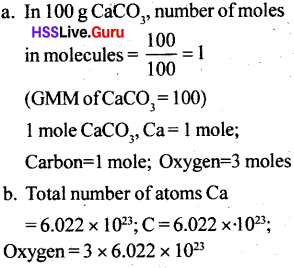Question 16.
Fill the blanks in the given table.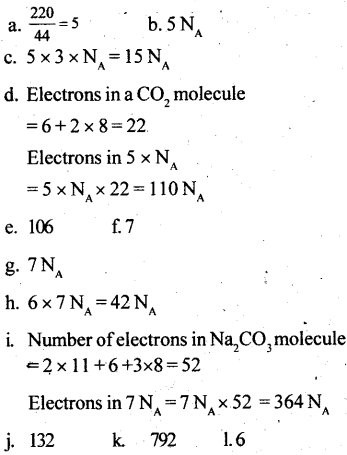Long Answer Type Questions (Spore 4)

Question 17.
See CO2 gas is taken in a cylinder provided with a piston. The cylinder is dipped in hot water.
a. What happens to the movement of CO2 molecules?
b. What change do you expect in the position of the piston?
c. What is the relation between temperature and the volume of a gas?
d State this gas law.
a. As the temperature increase, the energy of molecules increases. This increases the speed of the motion of molecules.
b. Piston is pulled in the upward direction. So piston moves upward.
c. As temperature increases, volume increases.
d. At constant pressure, the volume of a definite mass of gas is directly proportional to the temperature in Kelvin scale. (Charles law)Question 18.
The molecular formula of ammonium sulfate is (NH4)2SO4.
a. Find the gram molecular mass (GMM) of ammonium sulfate.
b. Calculate the number of molecules and atoms in 1.32g of ammonium sulfate.
a. GMM of (NH4)2SO4
= (14+4) × 2 + 32 + 4 × 16 = 36 + 32 + 64
= 132 g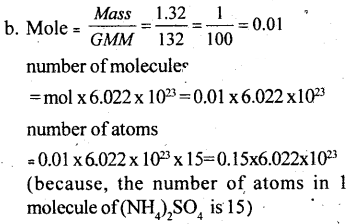Question 19.
Fill in the blanks of the table given below.Question 20.
See the diagram given below.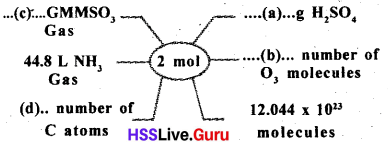a. 196g
b. 2 × 6.022 × 1023
c. 2 GMM
d. 2 × 6.022 × 1023

Question 21.
Write in pairs, equal number of atoms from those given below,
a. 2g Hydrogen
b. 16 g Oxygen
c. 14 g Nitrogen
d. 8 g HeliumQuestion 22.
Certain compounds and its masses are given,
i) 68 g NH3
ii) 28 g N2
iii) 9 g H2O
iv) 128 g O2
a. Which of these compounds have equal number of molecules?
b. How many molecules are there?
c. How many atoms are there in 9 g of water?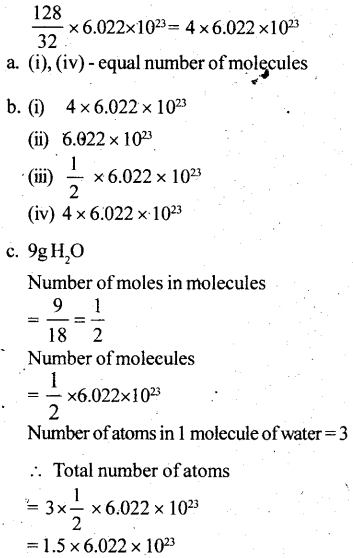Question 23.
368 g NO 2gas is given. Find the answers of each one given below,
a. GMM of NO2
b. Number of moles of molecules of 368 g NO2
c. Number of molecules
d. Number of atoms
e. Volume in STP
a. GMM of NO2
= 14 + 2 × 16 = 46 g

b. Number of mole = $$\frac { 368 }{ 46 }$$ = 8

c. Number of molecules = Number of moles × NA
= 8 × 6.022 × 1023

d. Number of atoms = Number of atoms in one molecule × Number of molecules
= 3 × 8 × 6.022 × 1023 = 24 × 6.022 × 1023

e. Volume in STP = Number of moles × 22.4
L = 8 × 22.4 L = 179.2 LQuestion 24.
Find out the GMMofthe following. Also find out the total number of atoms.
a. 20 g Nitrogen (H2)
b. 88.75 g Chlorine (Cl2)
c. 4 g Calcium (Ca2) ,
d. 7.75 g phosphorus (p4)
(H = 1, Cl =35.5, Ca =40, P=31)
a. 20 g of hydrogen (H2): GMM = $$\frac { 20 }{ 2 }$$ = 10
Number of molecules = 10 × 6.022 × 1023
Total number of atoms = 2 × 10 × 6.022 × 1023
= 20 × 6.022 × 1023

b. 88.75g of chlorine
Number of GMM = $$\frac { 88.75 }{ 71 }$$ = 1.25
Number of molecules = 1.25 × 6.022 × 1023
Total number of atoms = 2 × 1.25 × 6.022 × 1023
= 2.5 × 6.022 × 1023

c. 4 g Calcium (Ca)
Number of GMM = $$\frac { 4 }{ 40 }$$ = $$\frac { 1 }{ 10 }$$ = 0.1
Number of molecules = 0.1 × 6.022 × 1023
Total number of atoms = 1 × 0.1 × 6.022 × 1023
= 0.1 × 6.022 × 1023

d. 7.75 g Phosphorus Number of GMM = $$\frac { 7.75 }{ 31 }$$ = $$\frac { 1 }{ 4 }$$ = 0.25
Number of molecules = 0.25 × 6.022 × 1023